# Neoclassical Drift-MHD Equations

As before, we adopt the definitions (4.1)–(4.6) for the constants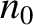,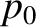,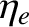,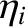,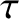, and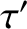that parameterize the equilibrium plasma density, pressure, and temperature, and their gradients, at the rational surface. We also make the simplifying assumption that perturbed electron and ion temperature profiles in the inner region are functions of the perturbed electron number density profile. Our neoclassical fluid equations, (2.370)–(2.374), reduce to the following set of neoclassical drift-MHD fluid equations: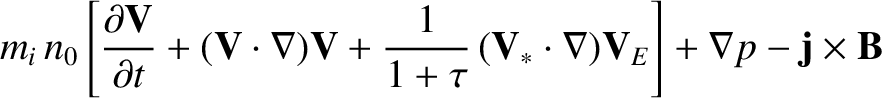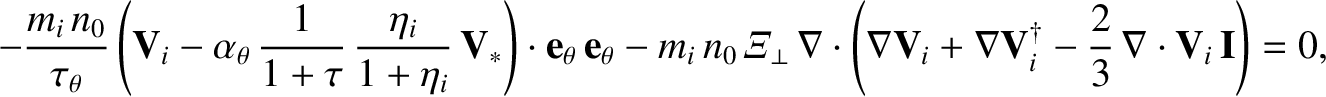(11.1)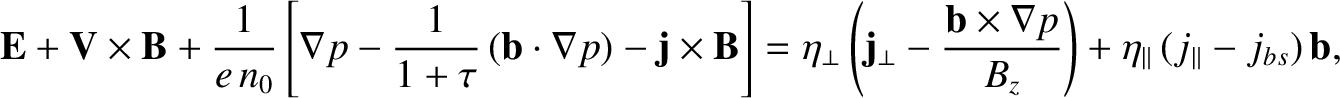(11.2)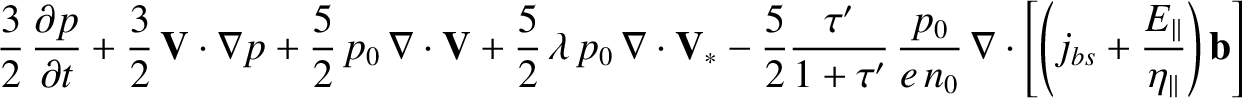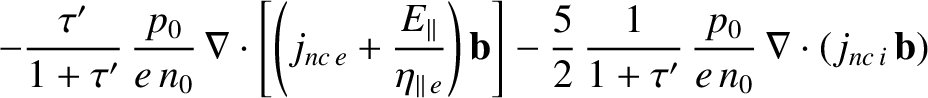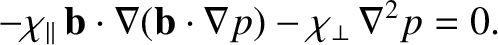(11.3)

Here, the E-cross-B, diamagnetic, MHD, and ion fluid velocities,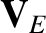,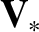,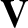, and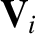, respectively, are defined in Equations (4.10)–(4.13). Moreover, the dimensionless parameter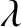, the ion perpendicular momentum diffusivity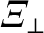, the parallel energy diffusivity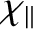, and the perpendicular energy diffusivity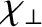, are defined in Equations (4.16)–(4.19). Finally,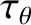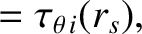(11.4)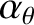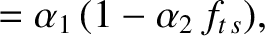(11.5)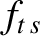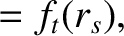(11.6)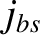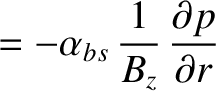(11.7)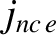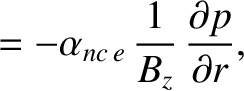(11.8)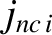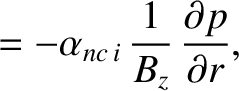(11.9)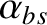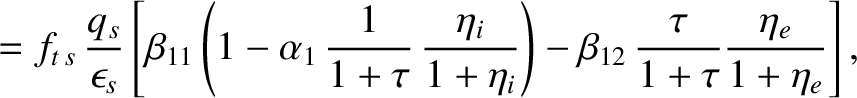(11.10)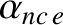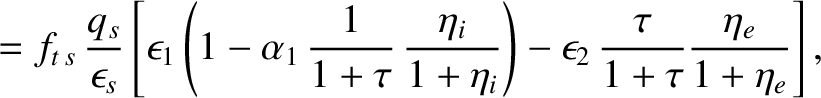(11.11)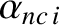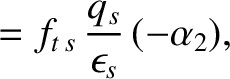(11.12)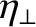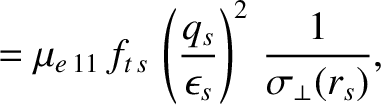(11.13)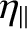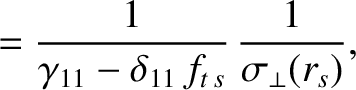(11.14)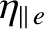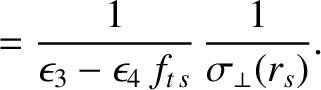(11.15)

Here,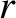is a radial cylindrical coordinate,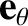is a unit vector in the poloidal direction (see Section 3.2),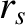is the minor radius of the rational surface,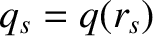is the safety-factor at the rational surface [see Equation (3.2)],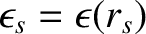is the inverse aspect-ratio at the rational surface [see Equation (3.18)], the ion neoclassical poloidal flow-damping time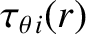is defined in Equation (2.332), the fraction of trapped particles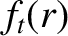is defined in Equation (2.202), the dimensionless neoclassical parameters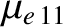,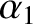,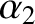,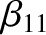,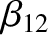,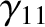,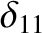,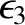, and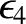are defined in Equations (2.209), (2.217), (2.218), (2.243), (2.244), (2.247), (2.251), (2.347), and (2.348), respectively, and the plasma perpendicular electrical conductivity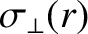is defined in Equation (2.41). As before,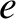is the magnitude of the electron charge,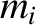the ion mass,the equilibrium electron number density at the rational surface,the equilibrium total plasma pressure at the rational surface,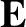the electric field-strength,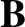the magnetic field-strength,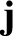the current density,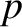the total plasma pressure, and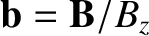. Finally,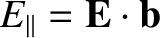,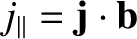, and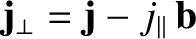.

If we compare Equation (11.1) with its non-neoclassical concomitant, (4.7), then we can see that the former equation contains an additional term that is due to the action of ion neoclassical poloidal flow damping. The term in question involves, which is the ion neoclassical poloidal flow-damping time at the rational surface. If we compare Equation (11.2) with its non-neoclassical concomitant, (4.8), then we can see that the former equation contains an additional term that is due to the action of the bootstrap current. The term in question involves, which is the parallel bootstrap current density at the rational surface. Finally, if we compare Equation (11.3) with its non-neoclassical concomitant, (4.9), then we can see that the former equation contains many additional terms that are due to neoclassical parallel momentum and heats flows.